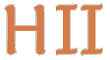# Hilbert II

 English Deutsch
 introduction news mathematics QEDEQ planning download glossary development prototype links contact site map
Planning

The basic concept gives a first impression of the planning for this project.

The planning for this project can be seen more clearly if we focus on the mathematical contents and describe the progress on that basis. With common mathematical practice in mind, the set theory used in Hilbert II is not ZFC but MK (an impredicative NBG extension). Our mathematical basis text is E. J. Lemmon's wonderful Introduction to Axiomatic Set Theory.

We started a script about axiomatic set theory. This script is written in an XML format that includes LaTeX texts and formulas. The document format is called QEDEQ. During the completion of the set theory script the QEDEQ format will be adopted and extended to be suitable for formal correct notations and proofs. The parser for reading QEDEQ documents and creating an appropriate Java object is developed simultaneously. Furthermore the generation of LaTeX files should always be possible. For our current script see: Axiomatic Set Theory.

We identified the following goals.

 1. Emma syntax for basic set theory Our formal syntax is so rich, that the necessary notations and formulas of basic set theory can be expressed. 2. Mymble formal contents of basic set theory We have a set theory script that has all the axioms, definitions and propositions written in a formal language in it. For that reason the propositions are always written as formulas. 3. Fillyjonk basic set theory informal complete Our set theory script is complete. Every theorem has an informal proof as usual. We have a simple LaTeX document that looks like a common mathematical textbook about axiomatic set theory. Ideally the mathematical description texts are written in different detail levels and in the languages English and German. 4. Snork all formulas checkable There is a kernel, which could check QEDEQ module files like the set theory script on a syntactic basis. For example it should recognize, that a formula is not well formed if it was quantified twice over the same subject variable. The kernel still couldn't check a proof (that means it couldn't decide if a formula derives logically from others). 5. The Groke basic set theory formal complete We now have also formal proofs within the basic set theory script and are able to check them. 6. Little My logic documented The underlying logic is fully documented in the script Elements of Mathematical Logic.

Currently we have nearly fulfilled Emma and more than half of Mymble. We have completed Snork and must extend the kernel when we add further proof methods. The basis of Little My is finished too. We have some proofs so Fillyjonk is on its way.
Even The Gorke has begun - we have our first formal proofs in qedeq_sample3.xml and can check them with our application for correctness. And there is more: we have a script with the axioms and inference rule of first-order predicate calculus which contains the beginning propositions of propositional calculus inclusive formal proofs: see qedeq_formal_logic_v1.

After completion of all stages we will start a script about number theory and work our way down again.

If you want to participate in this project, don't hesitate to contact us.

update 2014-01-20 09:20:48+0100